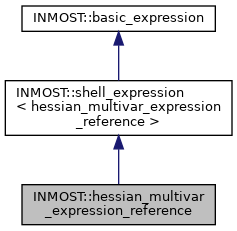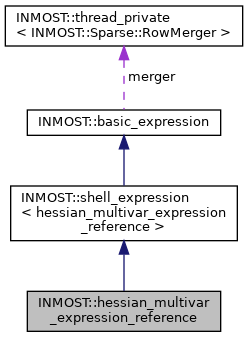INMOST A toolkit for distributed mathematical modeling
INMOST::hessian_multivar_expression_reference Class Reference
Inheritance diagram for INMOST::hessian_multivar_expression_reference:[legend]
Collaboration diagram for INMOST::hessian_multivar_expression_reference:[legend]

## Public Member Functions

hessian_multivar_expression_reference (INMOST_DATA_REAL_TYPE &_value, Sparse::Row *_entries, Sparse::HessianRow *_hentries)
Constructor, set links to the provided value and entries.

hessian_multivar_expression_reference (const hessian_multivar_expression_reference &other)
Copy constructor, sets links to the same reference of value and entries.

hessian_multivar_expression_reference (hessian_multivar_expression &other)
Copy constructor from hessian_multivar_expression, sets links to the same reference of value and entries.

__INLINE INMOST_DATA_REAL_TYPE GetValue () const
Retrieve value.

__INLINE void SetValue (INMOST_DATA_REAL_TYPE val)
Set value without changing derivatives.

__INLINE void GetJacobian (INMOST_DATA_REAL_TYPE mult, Sparse::RowMerger &r) const
Retrieve derivatives with multiplier into Sparse::RowMerger structure.

__INLINE void GetJacobian (INMOST_DATA_REAL_TYPE mult, Sparse::Row &r) const
Retrieve derivatives with multiplier into Sparse::Row structure.

__INLINE void GetHessian (INMOST_DATA_REAL_TYPE multJ, Sparse::Row &J, INMOST_DATA_REAL_TYPE multH, Sparse::HessianRow &H) const

__INLINE INMOST_DATA_REAL_TYPE GetDerivative (INMOST_DATA_ENUM_TYPE index) const

__INLINE multivar_expression GetVariable (INMOST_DATA_ENUM_TYPE index)

__INLINE hessian_multivar_expression_referenceoperator= (INMOST_DATA_REAL_TYPE pvalue)

__INLINE hessian_multivar_expression_referenceoperator= (basic_expression const &expr)

__INLINE hessian_multivar_expression_referenceoperator= (multivar_expression_reference const &other)

__INLINE hessian_multivar_expression_referenceoperator= (multivar_expression const &other)

__INLINE hessian_multivar_expression_referenceoperator= (hessian_multivar_expression_reference const &other)

__INLINE hessian_multivar_expression_referenceoperator= (hessian_multivar_expression const &other)

__INLINE Sparse::RowGetRow ()

__INLINE Sparse::HessianRowGetHessianRow ()

__INLINE const Sparse::RowGetRow () const

__INLINE const Sparse::HessianRowGetHessianRow () const

__INLINE hessian_multivar_expression_referenceoperator+= (basic_expression const &expr)

__INLINE hessian_multivar_expression_referenceoperator-= (basic_expression const &expr)

__INLINE hessian_multivar_expression_referenceoperator*= (basic_expression const &expr)

__INLINE hessian_multivar_expression_referenceoperator/= (basic_expression const &expr)

__INLINE hessian_multivar_expression_referenceoperator+= (INMOST_DATA_REAL_TYPE right)

__INLINE hessian_multivar_expression_referenceoperator-= (INMOST_DATA_REAL_TYPE right)

__INLINE hessian_multivar_expression_referenceoperator*= (INMOST_DATA_REAL_TYPE right)

__INLINE hessian_multivar_expression_referenceoperator/= (INMOST_DATA_REAL_TYPE right)

bool check_nans () const

bool check_infs () constPublic Member Functions inherited from INMOST::shell_expression< hessian_multivar_expression_reference >
virtual __INLINE INMOST_DATA_ENUM_TYPE GetCount () const

operator hessian_multivar_expression_reference & ()

operator const hessian_multivar_expression_reference & () constStatic Protected Attributes inherited from INMOST::basic_expression